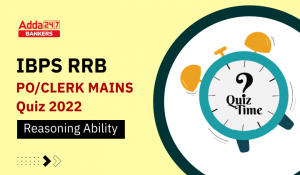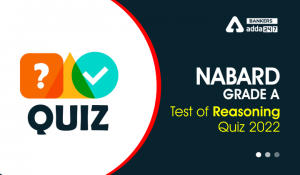Latest Banking jobs   »

# Reasoning Ability Quiz For RBI Grade B/ ECGC PO/ SIDBI Grade A Prelims 2022- 11th April

Directions (1-5): In these questions, relationship between different elements is show in the statements. The statements are followed by conclusions. Study the conclusions based on the given statements and select the appropriate answer:

(a) If only conclusion I follows.
(b) If only conclusion II follows.
(c) If either conclusion I or II follows
(d) If neither conclusion I nor II follows.
(e) If both conclusions I and II follow.

Q1. Statements: W≥Q>O=P>X=V<M=J
Conclusion I: V<W
II: V=W

Q2. Statements: Q>J≥L=C<B=E≥M>S=D
Conclusion I: L≥S
II: S<L

Q3. Statements: R≥E<V≤B≤N<S<O>Y>X
Conclusion I: S>E
II: O>R

Q4. Statements: U>R=S, V>W=F, S≤T≥V
Conclusion I: R≤V
II: R>V

Q5. Statements: T<J=K>L, N>M>R, R=T
Conclusion I: K>R
II: M>T

Directions (6-10): In these questions, relationships between elements is shown in the statements. These statements are followed by two conclusions. Give answer

(a) If only conclusion I follows.
(b) If only conclusion II follows.
(c) If either conclusion I or conclusion II follows.
(d) If neither conclusion I nor conclusion II follows.
(e) If both conclusions I and II follow.

Q6. Statements: G≤F=L≤J; J≤K=H
Conclusions: I. H=G
II. G<H

Q7. Statements: P<R<S<T>U
Conclusions: I. U<R
II. T>P

Q8. Statements: T>U≥V≥W; X<Y=W>Z
Conclusions: I. Z>U
II. W<T

Q9. Statements: K<L<M<N; M<O<P
Conclusions: I. P>K
II. N>O

Q10. Statements: B<A<C; A>D≤E
Conclusions: I. B≤E
II. C>E

Directions (11-15): In the following questions, the symbols #, %, \$, @ and © are used with the following meaning as illustrated below.
‘P # Q’ means ‘P is not smaller than Q’.
‘P % Q’ means ‘P is neither smaller than nor equal to Q’.
‘P \$ Q’ means ‘P is neither greater than nor equal to Q’.
‘P @ Q’ means ‘P is neither greater than nor smaller than Q’.
‘P © Q’ means ‘P is not greater than Q’.
Now, in each of the following questions assuming the given statements to be true, find which of the three Conclusions I, II and III given below them is/are definitely true and give your answer accordingly.

Q11. Statements: K%R@M, U©Q\$R, Q%T
Conclusions:
I. R%U
II. T#U
III. M%K
(a) Only I and III is true
(b) Only II and I is true
(c) Only II and III is true
(d) Only I is true
(e) None is true

Q12. Statements: D©G@ H, A%E#H, B\$D
Conclusions:
I. B%A
II. E#D
III. G%E
(a) Only III and II is true
(b) Only II
(c) Only III is true
(d) Either I or III true
(e) None of these

Q13. Statements: Z#A%C, G©Z, C#B\$H
Conclusions:
I.Z%B
II. H@Z
III. G#A
(a) Only I is true
(b) I, II and III are true
(c) Either I or II true
(d) Only II is true
(e) None of these

Q14. Statements: M\$P#Q, S%L#M, Q@W©R
Conclusions:
I. L#Q
II. R%P
III. W%L
(a) Only II and III is true
(b) Only I is true
(c) Either I or III true
(d) Only II
(e) None of these

Q15. Statements: K%M@R, R#T%X, O\$X
Conclusions:
I. M#T
II.R\$O
III. M%O
(a) Only I is true
(b) Only I and III is true
(c) Only II is true
(d) All are true
(e) None of these

Solutions

S1. Ans.(a)
Sol. I: V<W(true)
II: V=W(False)

S2. Ans.(d)
Sol. I: L≥S (False)
II: S<L (False)

S3. Ans.(a)
Sol. I: S>E (True)
II: O>R (False)

S4. Ans.(c)
Sol. I: R≤V (False)
II: R>V (false)

S5. Ans.(e)
Sol. I: K>R (True)
II: M>T(True)

S6.Ans.(c)
Sol. I. I.H=G (False)
II.G<H(False)

S7.Ans.(b)
Sol. I. U<R (False)
II.T>P(True)

S8.Ans.(b)
Sol. I. Z>U (False)
II.W<T(True)

S9.Ans.(a)
Sol. I. P>K (True)
II. N>O(False)

S10.Ans.(d)
Sol. I. B≤E (False)
II.C>E(False)

S11. Ans.(d)
Sol. I. R%U(true)
II. T#U(false)
III. M%K(false)

S12. Ans.(b)
Sol. I. B%A(false)
II. E#D(true)
III. G%E(false)

S13. Ans.(a)
Sol. I.Z%B(true)
II. H@Z(false)
III. G#A(false)

S14. Ans.(c)
Sol. I. L#Q(false)
II. R%P(false)
III. W%L(false)

S15. Ans.(b)
Sol. I. M#T(True)
II.R\$O(false)
III. M%O(True)#### Congratulations!Download Hindu Review of October 2021: Free PDFIncorrect details? Fill the form again here

Download Hindu Review of October 2021: Free PDF

Thank You, Your details have been submitted we will get back to you.
•Reasoning Quizzes For IBPS RRB PO/Clerk ...
•Reasoning Ability Quiz For IBPS RRB PO C...
•Reasoning Ability Quiz For IBPS RRB PO C...
•Reasoning Ability Quiz For IBPS RRB PO C...
•Reasoning Ability Quiz For IBPS Clerk Pr...
•Reasoning Ability Quiz For NABARD Grade ...